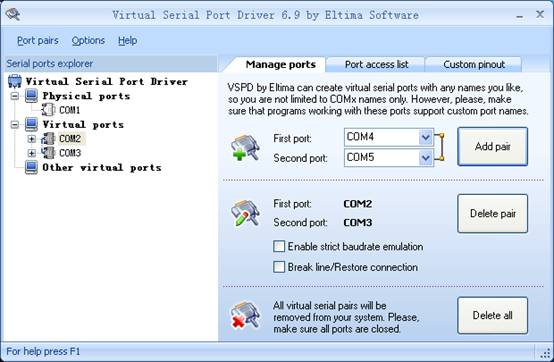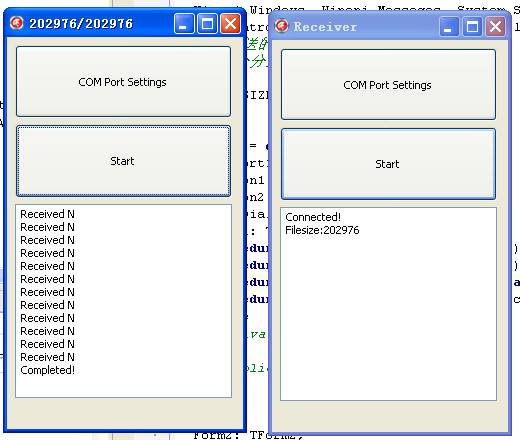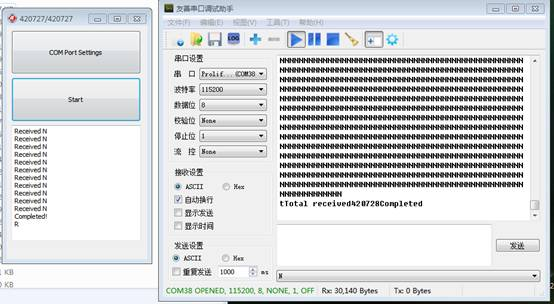# 设计一个文件传输的协议```procedure TForm2.ComPort1RxChar(Sender: TObject; Count: Integer);
var
s,t: String;

aSize,i:integer;
checksum:byte;
begin
if s[Count]='N' then
begin
if BytesSend<stream.size then
begin
fillchar(Buffer,BUFFERSIZE,0);
// 读取文件并取得实际读取出来的长度
//计算Checksum, 就是 Buffer 第一个到倒数第二个加起来要求为0
checksum:=0;
for i := 0 to BUFFERSIZE-3 do
begin
checksum:=checksum-Buffer[i];
end;
//放置Checksum
Buffer[BUFFERSIZE-2]:=checksum;
//放置顺序号
Buffer[BUFFERSIZE-1]:=Index;
inc(Index);
ComPort1.Write(Buffer,BUFFERSIZE);
{t:='';
for i := 0 to BUFFERSIZE-1 do
begin
t:=t+ IntToHex(Buffer[i],2)+' ';
end;
}
BytesSend:=BytesSend+aSize;
Form2.Caption:=IntToStr(BytesSend)+'/'+IntToStr(stream.size);
Form2.Refresh;
end
else
end;
//如果收到 R 就再次发送
if s[Count]='R' then
begin
ComPort1.Write(Buffer,BUFFERSIZE);
{     t:='';
for i := 0 to BUFFERSIZE-1 do
begin
t:=t+ IntToHex(Buffer[i],2)+' ';
end;
}
end;

end;```

Arduino代码如下 （Arduino我没有进行Index的校验）

```#include <SoftwareSerial.h>

#define BUFFERSIZE 16
#define TIMEOUT 3000UL
#define DEBUG (1)

//用来 DEBUG 的软串口，用额外的USB转串口线接在Pin11即可
SoftwareSerial mySerial(10, 11); // RX, TX

char buffer[BUFFERSIZE];

unsigned long filesize=0;  //文件大小
byte *p=(byte *)&filesize;
unsigned long total=0;

void setup() {
//收到的文件大小字节数，一共4位
byte c=0;
//每次收到的数值
byte r;

Serial.begin(115200);

if DEBUG {
// set the data rate for the SoftwareSerial port
mySerial.begin(115200);
mySerial.println("Hello, world?");
}

//如果没有收够4字节，则一直接收
while (c<4) {
while (Serial.available() > 0)
{
//比如文件大小为 0x10000, 那么上位机发出来的顺序是
// 00 00 01 00， 因此这里需要一个顺序的调换
*(p+c)=r;
c++;
if DEBUG {
mySerial.print(r);
mySerial.println("  ");
}
} //while (Serial.available() > 0)
} //while (c<4)

if DEBUG {
mySerial.print("filesize=");
mySerial.println(filesize);
}

//通知上位机继续发送
Serial.print('N');
}

void loop() {
byte buffer[BUFFERSIZE];
int i;
byte checksum;
Next:
//接收数据的字节数
int counter=0;
//接收超时的变量
unsigned long elsp=millis();
//如果接收数量小于缓冲区大小并且未超时，那么继续接收
while ((counter<BUFFERSIZE)&&(millis()-elsp<TIMEOUT))
{
while (Serial.available()>0)
{
if (DEBUG) {
// mySerial.print(buffer[counter],HEX);
// mySerial.print(" ");
// mySerial.println(counter,HEX);
}
counter++;
} //while
}
//如果接收数量不足退出上面的循环
if (counter!=BUFFERSIZE) {
//通知上位机重新发送
Serial.print("R");
if DEBUG {
mySerial.print("R");
}
}

//检查接收到的数据校验和
checksum=0;
for (i=0;i<BUFFERSIZE-1;i++)
{
checksum=checksum+buffer[i];
//Serial.print(buffer[i]);
//Serial.print("   ");
if DEBUG {
//mySerial.print(buffer[i]);
//mySerial.print("   ");
}
}
//校验失败通知上位机重新发送
if (checksum!=0)  {
Serial.print("R");
if DEBUG {
mySerial.print("R");
}
goto Next;
}

//如果当前收到的总数据小于文件大小，那么要求上位机继续发送
if (total<filesize)
{
Serial.print('N');
//有效值只有 BUFFERSIZE-2
total=total+BUFFERSIZE-2;
if DEBUG {
mySerial.print("N");
}
}
//否则停止
else {
if DEBUG {
mySerial.print(total);
}
while (1==1) {}
} //else

}```1.足够简单容易实现
2.对内存要求低

1.接收端只能等待外面过来的文件大小，如果这一步骤出现问题，那么后面都会乱掉，换句话说，如果你的通讯信道足够糟糕，那么整体还是不稳定；
2.效率不高，如果使用16位的Buffer,那么有效的数据只有 14字节，14 /16=87.5%。资料上说Uno 的串口默认是 64Byte，如果用这么大的Buffer，效率可以达到 （64-2）/64=96.9%。

Windows内部发送和接受代码和EXE（使用 128字节Buffer，速度挺快）